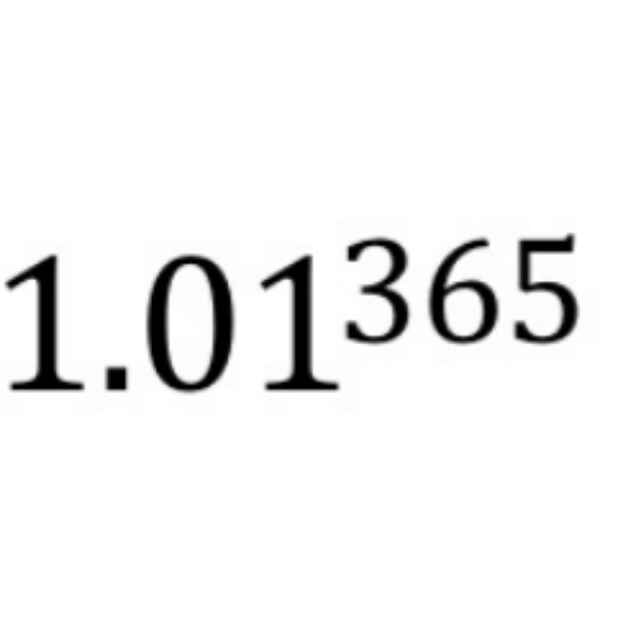# 权重初始化最佳实践• 因为对权重$w$的大小和正负缺乏先验，所以应初始化在0附近，但不能为全0或常数，所以要有一定的随机性，即数学期望$E(w)=0$
• 因为梯度消失和梯?#32570;?#28856;，权重不易过大或过小，所以要对权重的方差$Var(w)$有所控制

• 深度神经网络的多层结构中，每个激活层的输出对后面的层而言都是输入，所以我们希望不同激活层输出的方差相同，即$Var(a^{[l]})=Var(a^{[l-1]})$，这也就意味不同激活层输入的方差相同，即$Var(z^{[l]})=Var(z^{[l-1]})$
• 如果忽略激活函数，前向传播和反向传播可以看成是权重矩阵（转置）的连续相乘。数值太大，前向时可能陷入饱和区，反向时可能梯?#32570;?#28856;，数值太小，反向时可能梯度消失。所以初始化时，权重的数值范围（方差）应考虑到前向和后向两个过程# 期望与方差的相关性质

$Var(X) = E(X^2) - (E(X))^2$

$Cov(X, Y) = 0$

\begin{align} Cov(X, Y) &= E((X-E(X))(Y-E(Y))) \\ &= E(XY)-E(X)E(Y) =0 \end{align}

\begin{aligned} \operatorname{Var}(X+Y) &=E\left((X+Y)^{2}\right)-(E(X+Y))^{2} \\ &=E\left(X^{2}+Y^{2}+2 X Y\right)-(E(X)+E(Y))^{2} \\ &=\left(E\left(X^{2}\right)+E\left(Y^{2}\right)+2 E(X Y)\right)-\left((E(X))^{2}+(E(Y))^{2}+2 E(X) E(Y)\right) \\ &=\left(E\left(X^{2}\right)+E\left(Y^{2}\right)+2 E(X) E(Y)\right)-\left((E(X))^{2}+(E(Y))^{2}+2 E(X) E(Y)\right) \\ &=E\left(X^{2}\right)-(E(X))^{2}+E\left(Y^{2}\right)-(E(Y))^{2} \\ &=\operatorname{Var}(X)+\operatorname{Var}(Y) \end{aligned}

\begin{aligned} \operatorname{Var}(X Y) &=E\left((X Y)^{2}\right)-(E(X Y))^{2} \\ &=E\left(X^{2}\right) E\left(Y^{2}\right)-(E(X) E(Y))^{2} \\ &=\left(\operatorname{Var}(X)+(E(X))^{2}\right)\left(\operatorname{Var}(Y)+(E(Y))^{2}\right)-(E(X))^{2}(E(Y))^{2} \\ &=\operatorname{Var}(X) \operatorname{Var}(Y)+(E(X))^{2} \operatorname{Var}(Y)+\operatorname{Var}(X)(E(Y))^{2} \end{aligned}

# 全连接层方差分析

\begin{align}a_i^{[l-1]} &= f(z_i^{[l-1]}) \\z_i^{[l]} &= \sum_{j=1}^{fan\_in} w_{ij}^{[l]} \ a_j^{[l-1]}+b^{[l]} \\a_i^{[l]} &= f(z_i^{[l]})\end{align}

• 网络输入的每个元素$x_1,x_2,\dots$独立同分布
• 每层的权重随机初始化，同层的权重$w_{i1}, w_{i2}, \dots$独立同分布，且期望$E(w)=0$
• 每层的权重$w$和输入$a$随机初始化且相互独立，所以两者之积构成的随机变量$w_{i1}a_1, w_{i2}a_2, \dots$亦相互独立，且同分布；
• 根据上面的计算公式，同层的$z_1, z_2, \dots$独立同分布，同层的$a_1, a_2, \dots$也为独立同分布

\begin{align} Var(z) &=Var(\sum_{j=1}^{fan\_in} w_{ij} \ a_j) \\ &= fan\_in \times (Var(wa)) \\ &= fan\_in \times (Var(w) \ Var(a) + E(w)^2 Var(a) + Var(w) E(a)^2) \\ &= fan\_in \times (Var(w) \ Var(a) + Var(w) E(a)^2) \end{align}

# tanh下的初始化方法

$Var(a^{[l]}) = Var(z^{[l]}) = fan\_in \times Var(w) \times Var(a^{[l-1]})$

\begin{align} Var(a^{L}) = Var(z^{[L]}) &= n^{[L-1]} Var(w^{[L]}) Var(a^{[L-1]}) \\ &=\left[\prod_{l=1}^{L} n^{[l-1]} Var(w^{[l]})\right] {Var}(x) \end{align}

$\prod^{t} n^{[l-1]} Var(w^{[l]}) \\ \prod^{t} n^{[l]} Var(w^{[l]})$

## Lecun 1998

Lecun 1998年的paper Efficient BackProp ，在输入Standardization以及采用tanh激活函数的情况下，令$n^{[l-1]}Var(w^{[l]})=1$，即在初始化阶段?#20204;?#21521;传播过程每层方差保持不变，权重从如下高斯分布采样，其中第$l$层的$fan\_in = n^{[l-1]}$
$W \sim N(0, \frac{1}{fan\_in})$

## Xavier 2010

$W \sim N(0, \frac{2}{fan\_in + fan\_out})$

$Var(U(-n, n)) = \frac{n^2}{3}$

$n = \frac{\sqrt{6}}{\sqrt{fan\_in + fan\_out}}$

$W \sim U(-\frac{\sqrt{6}}{\sqrt{fan\_in + fan\_out}}, \frac{\sqrt{6}}{\sqrt{fan\_in + fan\_out}})$# ReLU/PReLU下的初始化方法

$Var(z)= fan\_in \times (Var(w) \ Var(a) + Var(w) E(a)^2)$\begin{align} Var(z) &= fan\_in \times (Var(w) \ Var(a) + Var(w) E(a)^2) \\ &= fan\_in \times (Var(w) (E(a^2) - E(a)^2)+Var(w)E(a)^2) \\ &= fan\_in \times Var(w) \times E(a^2) \end{align}

## He 2015 for ReLU

$Var(z^{[l]}) = fan\_in \times Var(w^{[l]}) \times E((a^{[l-1]})^2)$

\begin{align}Var(x) &= \int_{-\infty}^{+\infty}(x-0)^2 p(x) dx \\&= 2 \int_{0}^{+\infty}x^2 p(x) dx \\&= 2 E(\max(0, x)^2)\end{align}

\begin {align}Var(z^{[l]}) &= fan\_in \times Var(w^{[l]}) \times E((a^{[l-1]})^2) \\&= \frac{1}{2} \times fan\_in \times Var(w^{[l]}) \times Var(z^{[l-1]}) \end{align}

$\frac{1}{2} \times fan\_in \times Var(w^{[l]}) = 1 \\ Var(w) = \frac{2}{fan\_in}$

$W \sim N(0, \frac{2}{fan\_in})$

$W \sim N(0, \frac{2}{fan\_out})$## He 2015 for PReLU$\frac{1}{2} (1 + a^2) \times fan\_in \times Var(w^{[l]}) = 1 \\Var(w) = \frac{2}{(1 + a^2) fan\_in} \\W \sim N(0, \frac{2}{(1 + a^2) fan\_in}) \\W \sim N(0, \frac{2}{(1 + a^2) fan\_out})$

## caffe中的实现# 小结

?#38142;耍?#23545;深度神经网络权重初始化方法的介绍已告一段落。虽然因为BN层的提出，权重初始化可能已不再那么紧要。但是，对经典权重初始化方法经过一番剖析后，相信对神经网络运行机制的理解?#19981;?#26356;加深刻。

# 参考

posted @ 2019-11-21 21:42  shine-lee  阅读(...)  评论(... 编辑 收藏